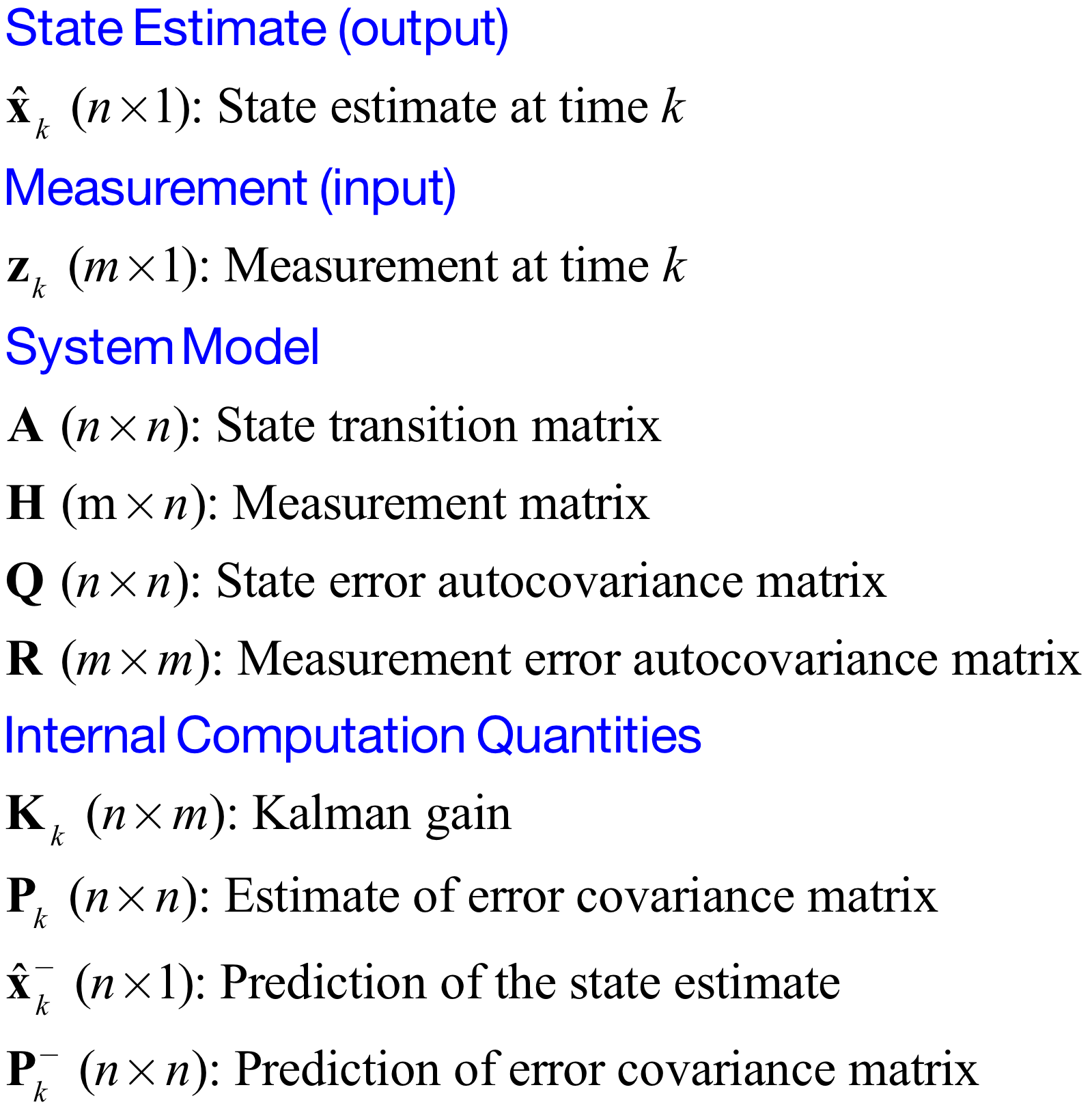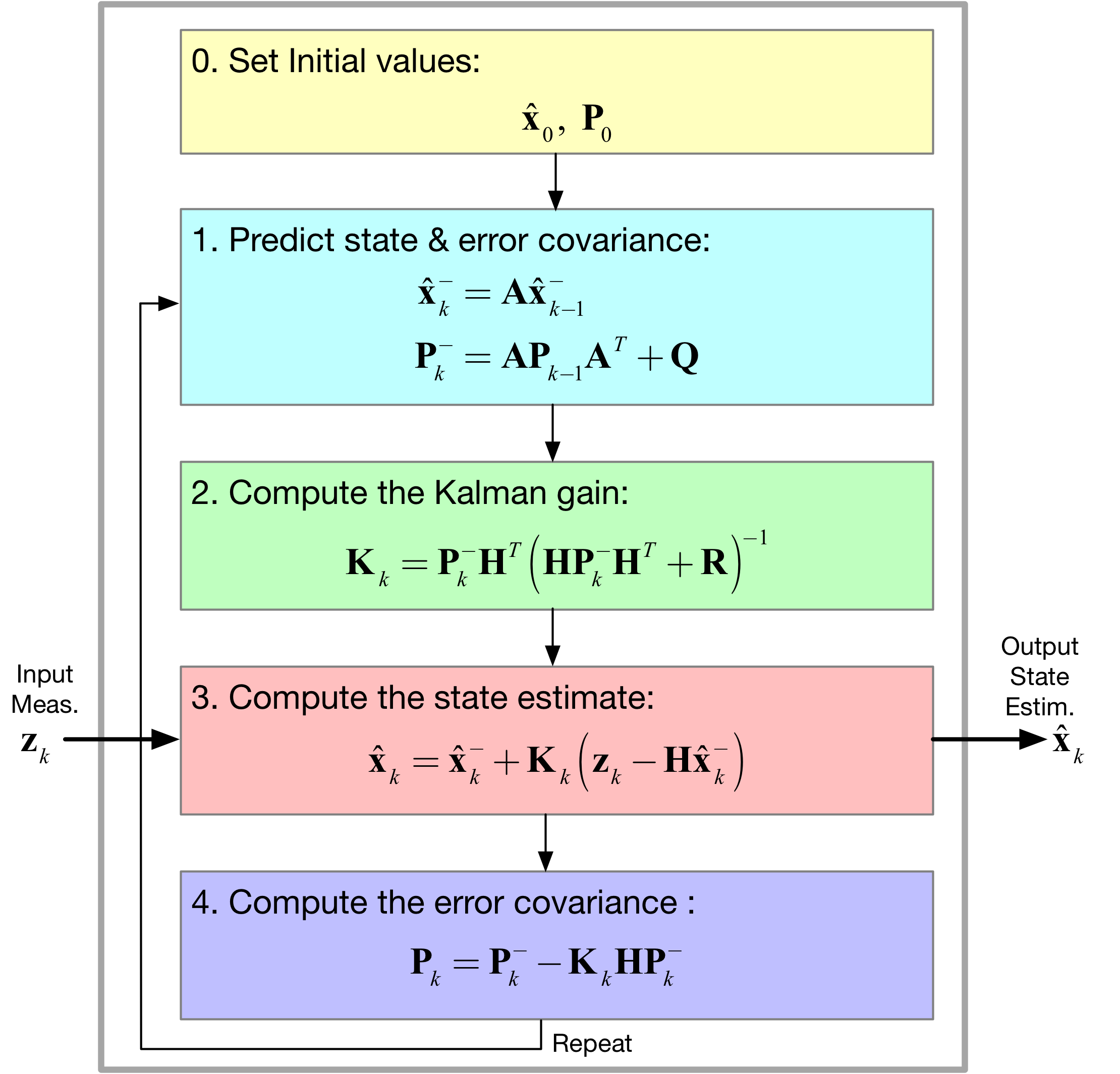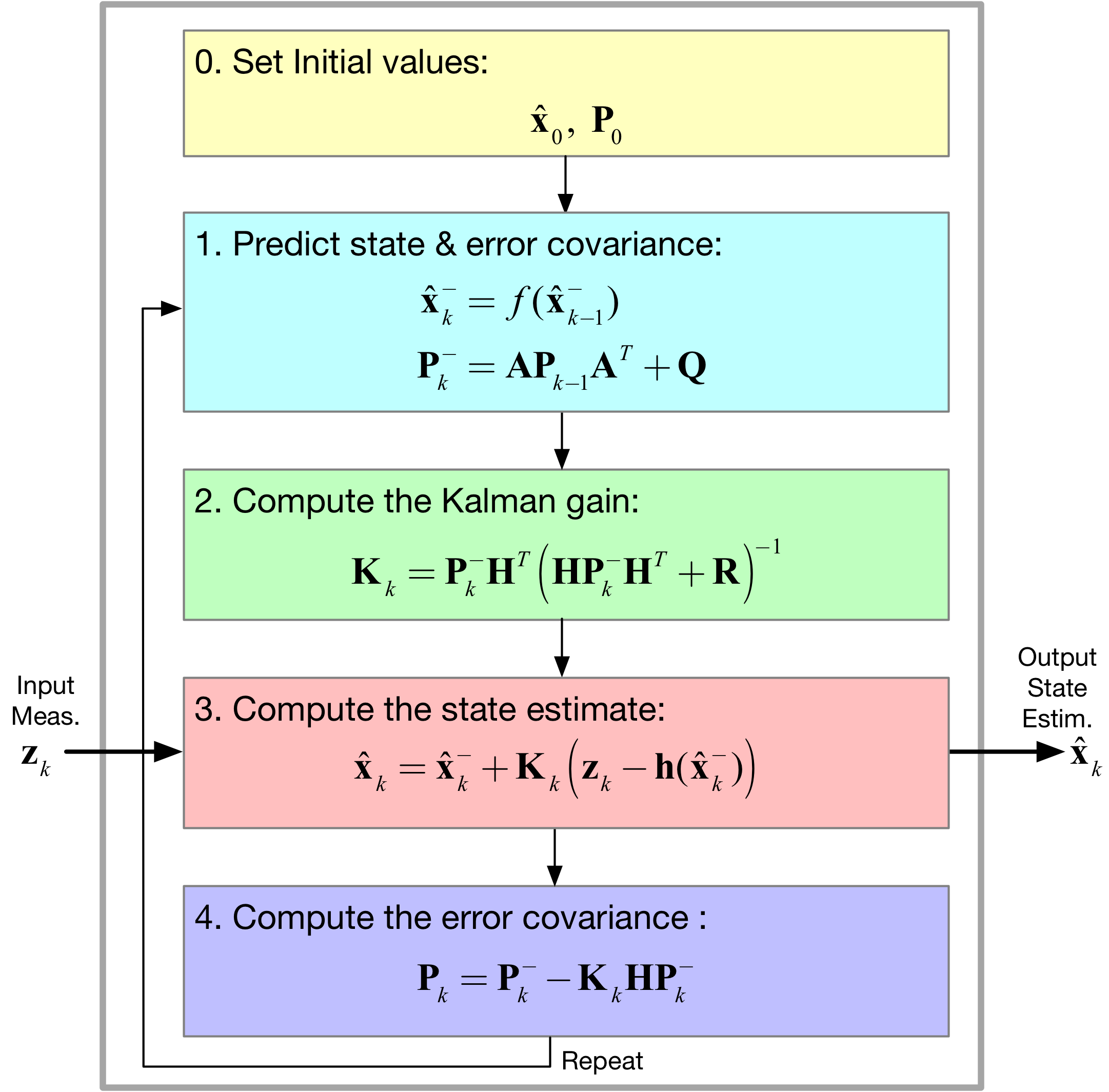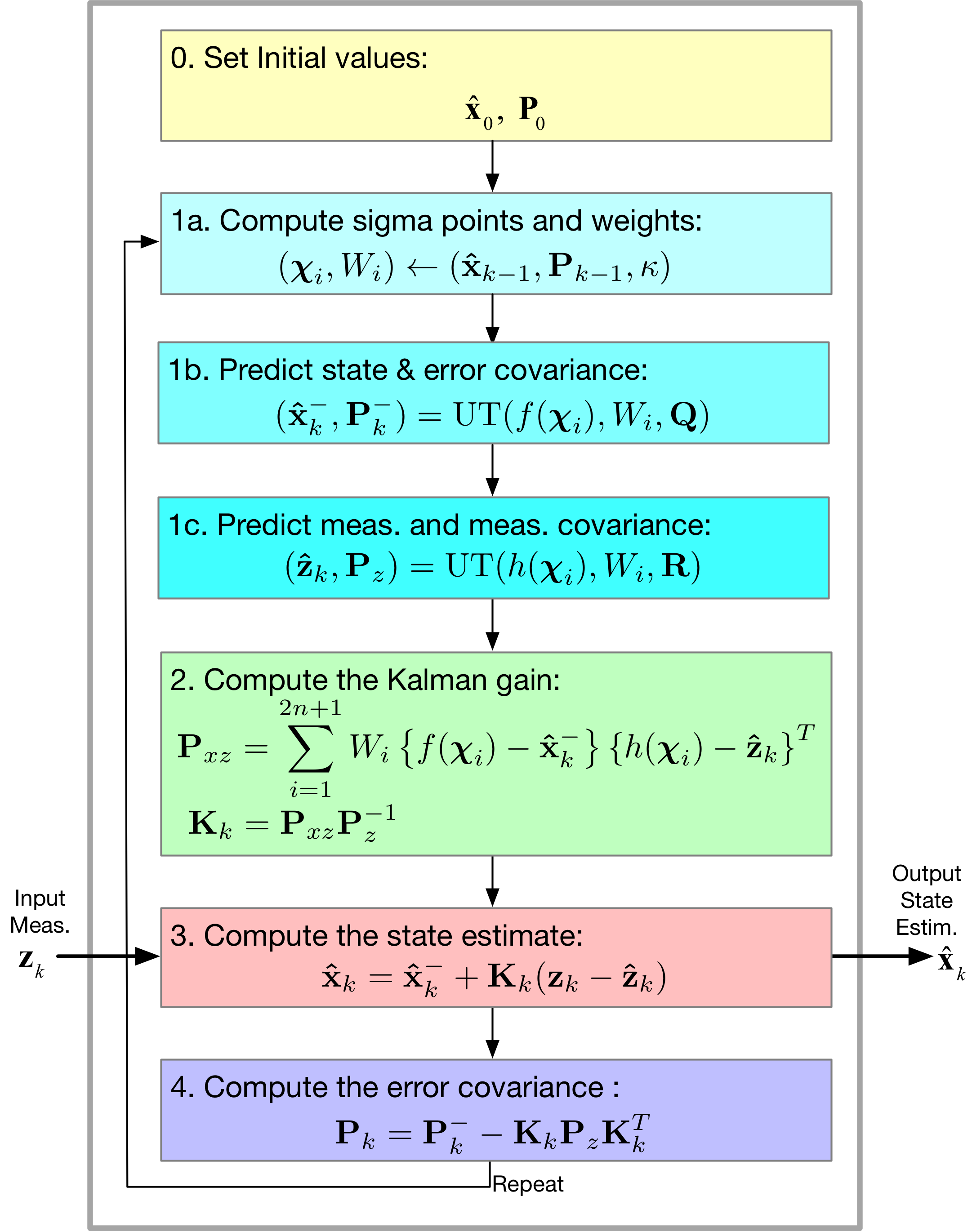:

%pylab inline
from inspect import getsource
import scipy.signal as signal
from IPython.display import Audio, display
from IPython.display import Image, SVG

Populating the interactive namespace from numpy and matplotlib

:

from gps_helper.smoothing import RecursiveAverage, MovingAverageFilter, LowPassFilter

:

pylab.rcParams['savefig.dpi'] = 100 # default 72
%config InlineBackend.figure_formats=['svg'] # SVG inline viewing


# Signal Filtering¶

:

figure(figsize=(6,5))
f0 =0.1
fs = 100
t = arange(0,20,1/fs)
s = sign(cos(2*pi*.1*t))
subplot(311)
title(r'0.1 Hz Squarewave with RC Lowpass')
ylabel(r'Signal - s')
plot(t,s)
grid()
subplot(312)
std_n = 0.4
r = s + std_n*randn(len(s))
plot(t,r)
ylabel(r's + n')
grid()
subplot(313)
f3 = 3*f0
a = exp(-2*pi*f3/fs)
rf = signal.lfilter([1-a],[1,-a],r)
plot(t,rf)
xlabel(r'Time (s)')
ylabel(r'LPF(s + n)')
grid()
tight_layout()# Measurement Filtering¶

:

print(getsource(RecursiveAverage))

class RecursiveAverage:
"""
Recursive average filter implementation.
"""

def __init__(self):
self.k = 0.0
self.x_k = 0

def next_sample(self, x):
"""
Process the next data sample.

:param x: Next data sample to compute average
:return: float
"""
self.k += 1.0
self.x_k = ((self.k - 1.) / self.k) * self.x_k + x / self.k
return self.x_k


:

print(getsource(MovingAverageFilter))

class MovingAverageFilter:
"""
Moving average filter implementation.
"""

def __init__(self, n):
"""
:param n: Number of samples to move the average over
"""
self.n = float(n)
self.x_k = [0.0 for ii in range(0, int(self.n))]

def next_sample(self, x):
"""
Process the next sample.

:param x: Next data sample to compute average
:return: float
"""
self.x_k[1:] = self.x_k[:-1]
self.x_k = x
return sum(self.x_k) / self.n


:

print(getsource(LowPassFilter))

class LowPassFilter:
"""
Low pass filter implementation.
"""

def __init__(self, alpha):
"""
Provide the alpha weighting value to initialize.
:param alpha: Weighting value
"""
self.alpha = alpha
self.prev = 0

def next_sample(self, x):
"""
Process the next sample.

:param x: Next sample value.
:return: float
"""
xlpf = self.alpha * self.prev + (1 - self.alpha) * x
self.prev = xlpf
return xlpf


:

Npts = 100
x = 5
v = 0.3*randn(Npts)
z = x + v
yravg = zeros_like(z)
ravg = RecursiveAverage()
y10 = zeros_like(z)
movavg10 = MovingAverageFilter(10)
y20 = zeros_like(z)
movavg20 = MovingAverageFilter(20)
lpf09 = LowPassFilter(0.9)
yfof09 = zeros_like(z)
for k, z_k in enumerate(z):
yravg[k] = ravg.next_sample(z_k)
y10[k] = movavg10.next_sample(z_k)
y20[k] = movavg20.next_sample(z_k)
yfof09[k] = lpf09.next_sample(z_k)
plot(arange(Npts),z,'r.',markersize=4)
plot(arange(Npts),yravg)
plot(arange(Npts),y10)
plot(arange(Npts),y20)
plot(arange(Npts),yfof09)
legend((r'Measurements',r'RecurAvg',r'MovAvg10',
r'MovAvg20',r'First-Order $\alpha=0.9$'))
ylabel(r'Filter Output')
xlabel(r'Index $n$')
title(r'Measurement Filtering a Noisy Constant')
grid();:

Npts = 100
n = arange(0,100)
x = 5*cos(2*pi*n/200)
v = 0.3*randn(Npts)
z = x + v
yravg = zeros_like(z)
ravg = RecursiveAverage()
y10 = zeros_like(z)
movavg10 = MovingAverageFilter(10)
y20 = zeros_like(z)
movavg20 = MovingAverageFilter(20)
lpf09 = LowPassFilter(0.9)
yfof09 = zeros_like(z)
for k, z_k in enumerate(z):
yravg[k] = ravg.next_sample(z_k)
y10[k] = movavg10.next_sample(z_k)
y20[k] = movavg20.next_sample(z_k)
yfof09[k] = lpf09.next_sample(z_k)
plot(arange(Npts),z,'r.',markersize=4)
plot(arange(Npts),yravg)
plot(arange(Npts),y10)
plot(arange(Npts),y20)
plot(arange(Npts),yfof09)
legend((r'Measurements',r'RecurAvg',r'MovAvg10',
r'MovAvg20',r'First-Order $\alpha=0.9$'))
ylabel(r'Filter Output')
xlabel(r'Index $n$')
title(r'Measurement Filtering Half Cycle Cosine')
grid();# Kalman Filtering Background¶

It was in 1960 that the Kalman filter was born. Today we are many many innovations beyond its humble beginnings.:

Image('figs/Kalman_variables.png',width='60%'):

Image('figs/Kalman_Filter.png',width='70%')


# Basic Examples from Kim¶

## Chapter 10.2: Estimating a Constant Voltage¶

:

class GetVoltage(object):
"""
A class for generating the battery voltage measurements

Mark Wickert February 2018
"""

def __init__ (self, batt_voltage = 14.4, dt = 0.2, sigma_w = 2):
"""
Initialize the object
"""
self.sigma_w = sigma_w
self.Voltage_set = batt_voltage

self.dt = dt

def measurement(self):
"""
Take a measurement
"""
w = 0 + self.sigma_w*random.randn(1)
z = self.Voltage_set + w
return z

:

class SimpleKalman(object):
"""
Kim Chapter 10.2 Battery voltage estimation with measurement noise

Python 3.x is assumed so the operator @ can be used for matrix multiply

Mark Wickert February 2018
"""

def __init__ (self, dt = 0.2, initial_state = 14, P = 6):
"""
Initialize the object
"""
self.dt = dt
self.A = array([])
self.H = array([])
# Process model covariance
self.Q = array([])
# Measurement model covariance
self.R = 4
self.x = array([[initial_state]])
# Error covariance initialize
self.P = P*eye(1)

def next_sample(self,z):
"""
Update the Kalman filter state by inputting a new
scalar measurement. Return the state array as a tuple
Update all other Kalman filter quantities
"""
xp = self.A @ self.x
Pp = self.A @ self.P @ self.A.T + self.Q

self.K = Pp @ self.H.T * inv(self.H @ Pp @ self.H.T + self.R)

self.x = xp + self.K @ (array([[z]] - self.H @ xp))
self.P = Pp - self.K @ self.H @ Pp

self.volt = self.x
return self.volt

:

dt = 0.1
t = arange(0,10+dt,dt)
Xsaved = zeros((len(t),2))
Zsaved = zeros(len(t))
Ksaved = zeros(len(t))
Psaved = zeros(len(t))

# Create objects for the simulation
GetVoltage1 = GetVoltage(14.0,dt,sigma_w = 2)
SimpleKalman1 = SimpleKalman(initial_state=14)

for k in range(len(t)):
z = GetVoltage1.measurement()
Xsaved[k,:] = SimpleKalman1.next_sample(z)
Zsaved[k] = z
Ksaved[k] = SimpleKalman1.K
Psaved[k] = SimpleKalman1.P

:

plot(t,Zsaved,'r.')
plot(t,Xsaved[:,0])
ylabel(r'Voltage (V)')
xlabel(r'Time (s)')
legend((r'Measured',r'Kalman Filter'),loc='best')
title(r'Voltage Estimation')
grid();:

subplot(211)
plot(t,Psaved)
ylabel(r'P')
xlabel(r'Time (s)')
title(r'Covariance ($\sigma_x^2$) as $P_k$')
grid();
subplot(212)
plot(t,Ksaved)
ylabel(r'K')
xlabel(r'Time (s)')
title(r'Kalman Gain $K_k$')
grid();
tight_layout():

plot(t,Ksaved)
ylabel(r'K')
xlabel(r'Time (s)')
grid();:

class GetPosVel(object):
"""
A class for generating position and velocity
measurements and truth values
of the state vector.

Mark Wickert May 2018
"""

def __init__ (self,pos_set = 0, vel_set = 80.0, dt = 0.1,
Q = [[1,0],[0,3]], R = [[10,0],[0,2]]):
"""
Initialize the object
"""
self.actual_pos = pos_set
self.actual_vel = vel_set

self.Q = array(Q)
self.R = array(R)
self.dt = dt

def measurement(self):
"""
Take a measurement
"""
# Truth position and velocity
self.actual_vel = self.actual_vel
self.actual_pos = self.actual_pos \
+ self.actual_vel*self.dt

# Measured value is truth plus measurement error
z1 = self.actual_pos + sqrt(self.R[0,0])*random.randn()
z2 = self.actual_vel + sqrt(self.R[1,1])*random.randn()
return array([[z1],[z2]])

:

class PosKalman(object):
"""
Position Estimation from Position and Velocity Measurements

Python 3.x is assumed so the operator @ can be used for matrix multiply

Mark Wickert May 2018
"""

def __init__ (self, Q, R, initial_state = [0, 20], dt = 0.1):
"""
Initialize the object
"""
self.dt = dt
self.A = array([[1, dt],[0,1]])
self.H = array([[1,0],[0,1]])
# Process model covariance
self.Q = Q
# Measurement model covariance
self.R = R
self.x = array([[initial_state],[initial_state]])
# Error covariance initialize
self.P = 5*eye(2)
# Initialize state
self.x = array([[0.0],[0.0]])

def next_sample(self,z):
"""
Update the Kalman filter state by inputting a new
scalar measurement. Return the state array as a tuple
Update all other Kalman filter quantities
"""
xp = self.A @ self.x
Pp = self.A @ self.P @ self.A.T + self.Q

self.K = Pp @ self.H.T * inv(self.H @ Pp @ self.H.T + self.R)

self.x = xp + self.K @ (z - self.H @ xp)
self.P = Pp - self.K @ self.H @ Pp
return self.x


### Run a Simulation¶

:

dt = 0.1
t = arange(0,10+dt,dt)
Xsaved = zeros((2,len(t)))
Zsaved = zeros((2,len(t)))
Psaved = zeros(len(t))
Vsaved = zeros(len(t))
# Save history of error covariance matrix diagonal
P_diag = zeros((len(t),2))

# Create objects for the simulation
Q = array([[1,0],[0,3]])
R = array([[10,0],[0,2]])
GetPos1 = GetPosVel(Q=Q,R=R,dt=dt)
PosKalman1 = PosKalman(Q,R, initial_state=[0,80])

for k in range(len(t)):
# take a measurement
z = GetPos1.measurement()
# Update the Kalman filter
Xsaved[:,k,None] = PosKalman1.next_sample(z)
Zsaved[:,k,None] = z
Psaved[k] = GetPos1.actual_pos
Vsaved[k] = GetPos1.actual_vel
P_diag[k,:] = PosKalman1.P.diagonal()

:

figure(figsize=(6,3.5))
plot(t,Zsaved[0,:]-Psaved,'r.')
plot(t,Xsaved[0,:]-Psaved)
xlabel(r'Time (s)')
ylabel(r'Position Error (m)')
title(r'Position Error: $x_1 - p_{true}$')
legend((r'Measurement Error',r'Estimation Error'))
grid();:

figure(figsize=(6,3.5))
plot(t,Zsaved[1,:]-Vsaved,'r.')
plot(t,Xsaved[1,:]-Vsaved)
xlabel(r'Time (s)')
ylabel(r'Velocity Error (m)')
title(r'Velocity Error: $x_2 - v_{true}$')
legend((r'Measurement Error',r'Estimation Error'))
grid();:

figure(figsize=(6,3.5))
plot(t,P_diag[:,0])
plot(t,P_diag[:,1])
title(r'Covariance Matrix $\mathbf{P}$ Diagonal Entries')
ylabel(r'Variance')
xlabel(r'Time (s) (given $T_s = 0.1$s)')
legend((r'$\sigma_p^2$ (m$^2$)',r'$\sigma_v^2$ (m$^4$)'))
grid();## Chapter 11.2 & 11.3: Estimating Velocity from Position¶

:

class GetPos(object):
"""
A class for generating position measurements as found in Kim

Mark Wickert December 2017
"""

def __init__ (self,Posp = 0, Vel_set = 80.0, dt = 0.1,
var_w = 10.0, var_v = 10.0):
"""
Initialize the object
"""
self.Posp = Posp
self.Vel_set = Vel_set
self.Velp = Vel_set

self.dt = dt

self.var_w = var_w
self.var_v = var_v

def measurement(self):
"""
Take a measurement
"""
# The velocity process noise
w = 0 + self.var_w*random.randn(1)
# The position measurement noise
v = 0 + self.var_v*random.randn(1)

# Update the position measurement
z = self.Posp + self.Velp*self.dt + v
# Also update the truth values of position and velocity
self.Posp = z - v
self.Velp = self.Vel_set + w
return z

:

class DvKalman(object):
"""
Kim Chapter 11.2 Velocity from Position Estimation

Python 3.x is assumed so the operator @ can be used for matrix multiply

Mark Wickert December 2017
"""

def __init__ (self,initial_state = [0, 20]):
"""
Initialize the object
"""
self.dt = 0.1
self.A = array([[1, self.dt],[0,1]])
self.H = array([[1,0]])
# Process model covariance
self.Q = array([[1,0],[0,3]])
# Measurement model covariance
self.R = 10
self.x = array([[initial_state],[initial_state]])
# Error covariance initialize
self.P = 5*eye(2)
# Initialize pos and vel
self.pos = 0.0
self.vel = 0.0

def next_sample(self,z):
"""
Update the Kalman filter state by inputting a new
scalar measurement. Return the state array as a tuple
Update all other Kalman filter quantities
"""
xp = self.A @ self.x
Pp = self.A @ self.P @ self.A.T + self.Q

self.K = Pp @ self.H.T * inv(self.H @ Pp @ self.H.T + self.R)

self.x = xp + self.K @ (array([[z]] - self.H @ xp))
self.P = Pp - self.K @ self.H @ Pp

self.pos = self.x
self.vel = self.x
return self.pos, self.vel


### Run a Simulation¶

:

dt = 0.1
t = arange(0,10+dt,dt)
Xsaved = zeros((len(t),2))
Zsaved = zeros(len(t))
Vsaved = zeros(len(t))

# Create objects for the simulation
GetPos1 = GetPos()
DvKalman1 = DvKalman()

for k in range(len(t)):
z = GetPos1.measurement()
# pos, vel = DvKalman1.update(z)

Xsaved[k,:] = DvKalman1.next_sample(z)
Zsaved[k] = z
Vsaved[k] = GetPos1.Velp

:

plot(t,Zsaved,'r.')
plot(t,Xsaved[:,0])
ylabel(r'Position (m)')
xlabel(r'Time (s)')
legend((r'Measured',r'Kalman Filter'),loc='best')
grid();:

plot(t,Vsaved,'r.')
plot(t,Xsaved[:,1])
ylabel(r'Velocity (m/s)')
xlabel(r'Time (s)')
legend((r'True Speed',r'Kalman Filter'),loc='best')
grid();## Chapter 11.4: Estimating Position from Velocity¶

:

class GetVel(object):
"""
A class for generating velocity measurements as found in Kim 11.4

Mark Wickert December 2017
"""

def __init__ (self,Pos_set = 0, Vel_set = 80.0, dt = 0.1, var_v = 10.0):
"""
Initialize the object
"""
self.Posp = Pos_set
self.Vel_set = Vel_set
self.Velp = Vel_set

self.dt = dt

self.var_v = var_v

def measurement(self):
"""
Take a measurement
"""
# The velocity measurement noise
v = 0 + self.var_v*random.randn(1)

# Also update the truth values of position and velocity
self.Posp += self.Velp*self.dt
self.Velp = self.Vel_set + v
z = self.Velp
return z

:

class IntKalman(object):
"""
Kim Chapter 11.4 Position from Velocity Estimation

Python 3.x is assumed so the operator @ can be used for matrix multiply

Mark Wickert December 2017
"""

def __init__ (self,initial_state = [0, 20]):
"""
Initialize the object
"""
self.dt = 0.1
self.A = array([[1, dt],[0,1]])
self.H = array([[0,1]])
# Process model covariance
self.Q = array([[1,0],[0,3]])
# Measurement model covariance
self.R = 10
self.x = array([[initial_state],[initial_state]])
# Error covariance initialize
self.P = 5*eye(2)
# Initialize pos and vel
self.pos = 0.0
self.vel = 0.0

def next_sample(self,z):
"""
Update the Kalman filter state by inputting a new scalar measurement.
Return the state array as a tuple
Update all other Kalman filter quantities
"""
xp = self.A @ self.x
Pp = self.A @ self.P @ self.A.T + self.Q

self.K = Pp @ self.H.T * inv(self.H @ Pp @ self.H.T + self.R)

self.x = xp + self.K @ (array([[z]] - self.H @ xp))
self.P = Pp - self.K @ self.H @ Pp

self.pos = self.x
self.vel = self.x
return self.pos, self.vel

:

dt = 0.1
t = arange(0,10+dt,dt)
Xsaved = zeros((len(t),2))
Zsaved = zeros(len(t))
Psaved = zeros(len(t))

# Create objects for the simulation
GetVel1 = GetVel()
IntKalman1 = IntKalman()

for k in range(len(t)):
z = GetVel1.measurement()

Xsaved[k,:] = IntKalman1.next_sample(z)
Zsaved[k] = z
Psaved[k] = GetVel1.Posp

:

plot(t,Zsaved,'r.')
plot(t,Xsaved[:,1])
ylabel(r'Velocity (m/s)')
xlabel(r'Time (s)')
legend((r'Measurements',r'Kalman Filter'),loc='best')
grid();:

plot(t,Xsaved[:,0])
plot(t,Psaved)
ylabel(r'Position (m)')
xlabel(r'Time (s)')
legend((r'True Position' ,r'Kalman Filter',),loc='best')
grid();## Chapter 11.5: Measuring Velocity with Sonar¶

:

# From scipy.io

:

class GetSonar(object):
"""
A class for playing back sonar altitude measurements as found in Kim 2.4
and later used in Kim 11.5

Mark Wickert December 2017
"""

def __init__ (self,):
"""
Initialize the object
"""
self.h = sonarD['sonarAlt'].flatten()
self.Max_pts = len(self.h)
self.k = 0

def measurement(self):
"""
Take a measurement
"""

h = self.h[self.k]
self.k += 1
if self.k > self.Max_pts:
print('Recycling data by starting over')
self.k = 0
return h

:

Nsamples = 500
t = arange(Nsamples)*.02
Xsaved = zeros(Nsamples)
Xmsaved = zeros(Nsamples)
Xksaved = zeros((Nsamples,2))

GetSonar1 = GetSonar()
MovAvgFilter1 = MovingAverageFilter(10)
DvKalman2 = DvKalman()
for k in range(Nsamples):
xm =GetSonar1.measurement()
Xmsaved[k] = xm
Xsaved[k] = MovAvgFilter1.next_sample(xm)
Xksaved[k,:] = DvKalman2.next_sample(xm)

:

plot(t,Xmsaved,'r.',markersize=4)
plot(t,Xsaved)
plot(t,Xksaved[:,0])
xlim([0,10])
ylabel(r'Altitude (m)')
xlabel(r'Time (s)')
legend((r'Measurement' ,r'Moving Average - 10',r'Kalman-pos'),loc='best')
grid();:

plot(t,Xksaved[:,1],'orange')
xlim([0,10])
ylabel(r'Velocity (m/s)')
xlabel(r'Time (s)')
legend((r'Kalman-vel',),loc='best')
grid();# Nonlinear Kalman Filter¶

## Chapter 14 Extended Kalman Filter (EKF)¶

Here we focus on:

• Replacing $$\mathbf{Ax}_k$$ with the nonlinearity $$f(\mathbf{x}_k)$$ and $$\mathbf{Hx}_k$$ with the nonlinearity $$\mathbf{h}(\mathbf{x}_k)$$
• How in the end the EKF linearizes the nonlinear model at each time step by using the Jacobian matrices $$\mathbf{A} \equiv \partial \mathbf{f}/\partial \mathbf{x}$$ and $$\mathbf{H} \equiv \partial \mathbf{h}/\partial \mathbf{x}$$
• Assembling the EKF algorithm from the Kalman filter foundation
• Finally, a Radar tracking example (Kim 14.4):

Image('figs/EKF_Filter.png',width='70%')

:

class GetRadar(object):
"""
A class for generating radar slant range measurements as found in Kim 14.4

Mark Wickert December 2017
"""

def __init__ (self,Pos_set = 0, Vel_set = 80.0, Alt_set = 1000, dt = 0.1,
var_Vel = 25.0, var_Alt = 100):
"""
Initialize the object
"""
self.Posp = Pos_set
self.Vel_set = Vel_set
self.Alt_set = Alt_set

self.dt = dt

self.var_Vel = var_Vel
self.var_Alt = var_Alt

def measurement(self):
"""
Take a measurement
"""
# The velocity process with uncertainty
vel = self.Vel_set + sqrt(self.var_Vel)*random.randn(1)
# The altitude process with uncertainty
alt = self.Alt_set + sqrt(self.var_Alt)*random.randn(1)

# New position
pos = self.Posp + vel*dt

# Slant range measurement noise
v = 0 + pos*0.05*random.randn(1)
# The slant range
r = sqrt(pos**2 + alt**2) + v
self.Posp = pos
return r

:

class RadarEKF(object):
"""
Kim Chapter 14.4 Radar Range Tracking

Python 3.x is assumed so the operator @ can be used for matrix multiply

Mark Wickert December 2017
"""

def __init__ (self, dt=0.05, initial_state = [0, 90, 1100]):
"""
Initialize the object
"""
self.dt = dt
self.A = eye(3) + dt*array([[0, 1, 0], [0, 0, 0], [0, 0, 0]])
# Process model covariance
self.Q = array([[0, 0, 0], [0, 0.001, 0], [0, 0, 0.001]])
# Measurement model covariance
self.R = array([])
self.x = array(initial_state)
# Error covariance initialize
self.P = 10*eye(3)
# Initialize pos and vel
self.pos = 0.0
self.vel = 0.0
self.alt = 0.0

def next_sample(self,z):
"""
Update the Kalman filter state by inputting a new scalar measurement.
Return the state array as a tuple
Update all other Kalman filter quantities
"""
H = self.Hjacob(self.x)
xp = self.A @ self.x
Pp = self.A @ self.P @ self.A.T + self.Q

self.K = Pp @ H.T * inv(H @ Pp @ H.T + self.R)

self.x = xp + self.K @ (array([z - self.hx(xp)]))
self.P = Pp - self.K @ H @ Pp

self.pos = self.x
self.vel = self.x
self.alt = self.x
return self.pos, self.vel, self.alt

def hx(self,xhat):
"""
State vector predicted to slant range
"""
zp = sqrt(xhat**2 + xhat**2)
return zp

def Hjacob(self,xp):
"""
Jacobian used to linearize the measurement matrix H
given the state vector
"""
H = zeros((1,3))

H[0,0] = xp/sqrt(xp**2 + xp**2)
H[0,1] = 0
H[0,2] = xp/sqrt(xp**2 + xp**2)
return H


### Run a Simulation¶

:

Nsamples = 500
dt = 0.05
t = arange(Nsamples)*dt
Nsamples = len(t)
XsavedEKF = zeros((Nsamples,3))
XmsavedEKF = zeros(Nsamples)
ZsavedEKF = zeros(Nsamples)

for k in range(Nsamples):
XmsavedEKF[k] = xm
ZsavedEKF[k] = norm(XsavedEKF[k])

:

figure(figsize=(6,5))
subplot(311)
plot(t,XsavedEKF[:,1])
title(r'Extended Kalman Filter States')
ylabel(r'Vel (m/s)')
xlabel(r'Time (s)')
grid();
subplot(312)
plot(t,XsavedEKF[:,2])
ylabel(r'Alt (m)')
xlabel(r'Time (s)')
grid();
subplot(313)
plot(t,XsavedEKF[:,0])
ylabel(r'Pos (m)')
xlabel(r'Time (s)')
grid();
tight_layout():

plot(t,XmsavedEKF,'r.',markersize=4)
plot(t,ZsavedEKF)
ylabel(r'Slant Range (m)')
xlabel(r'Time (s)')
legend((r'Measured',r'EKF Estimated'),loc='best')
grid();## Chapter 15 Unscented KalmanFilter (UKF)¶

Here we focus on:

• The unscented transformation algorithm
• The estimation of the mean and convariance matrix asscociated with the joint pdf of $$y = f(x)$$, where in general $$x$$ and $$y$$ are vectors, e.g., the state and transformed state
• Assembling the UKF algorithm from the original KF, but with new and substitute equations due to sigma point generation and transformed sigma points
• Finally, a Radar tracking example (continuation) from Kim 15.4:

Image('figs/UKF_Filter.png',width='70%')


### Preliminaries¶

First review how to slice a 2D array into a 2D column or row vector using a third argument set to None. Note this approach works for both right-side and left-side calculations. You know you have done something wrong when you receive a numpy broadcasting error.

:

A = arange(1,21).reshape(4,5)
print('# A 4 x 5 matrix:')
print(A)
print('# Slice out the second column as a 4x1 matrix')
print(A[:,1,None])
print('# Slice out the third row as a 1x5 matrix')
print(A[2,None,:])

# A 4 x 5 matrix:
[[ 1  2  3  4  5]
[ 6  7  8  9 10]
[11 12 13 14 15]
[16 17 18 19 20]]
# Slice out the second column as a 4x1 matrix
[[ 2]
[ 7]

]
# Slice out the third row as a 1x5 matrix
[[11 12 13 14 15]]

:

def SigmaPoints(xm, P, kappa):
"""
Calculate the Sigma Points of an unscented Kalman filter

Mark Wickert December 2017
Translated P. Kim's program from m-code
"""
n  = xm.size
Xi = zeros((n, 2*n+1))  # sigma points = col of Xi
W  = zeros(2*n+1)
Xi[:, 0, None] = xm
W = kappa/(n + kappa)

U = cholesky((n+kappa)*P)  # U'*U = (n+kappa)*P

for k in range(n):
Xi[:, k+1, None] = xm + U[k, None, :].T   # row of U
W[k+1] = 1/(2*(n+kappa))

for k in range(n):
Xi[:, n+k+1, None] = xm - U[k, None, :].T
W[n+k+1] = 1/(2*(n+kappa))

return Xi, W

:

def UT(Xi, W, noiseCov = 0):
"""
Unscented transformation

Mark Wickert December 2017
Translated P. Kim's program from m-code
"""
n, kmax = Xi.shape

xm = zeros((n,1))
for k in range(kmax):
xm += W[k]*Xi[:, k, None]

xcov = zeros((n, n))
for k in range(kmax):
xcov += W[k]*(Xi[:, k, None] - xm)*(Xi[:, k, None] - xm).T

xcov += noiseCov
return xm, xcov


### Verify that SigmaPoints() and UT() are working:¶

:

xm = array([,])
Px = 9*eye(2)
kappa = 2

Xi, W = SigmaPoints(xm,Px,kappa) # sigma points and weights
xAvg, xCov = UT(Xi, W) # estimate mean vector and covariance matrix using sigma points

:

print(Xi)
print(W)
print(xAvg)
print(xCov)

[[ 5. 11.  5. -1.  5.]
[ 5.  5. 11.  5. -1.]]
[0.5   0.125 0.125 0.125 0.125]
[[5.]
[5.]]
[[9. 0.]
[0. 9.]]

• The xAvg and xCon values match the original input values of mean and covariance
:

class RadarUKF(object):
"""
Kim Chapter 15.4 Radar Range Tracking UKF Version

Python 3.x is assumed so the operator @ can be used for matrix multiply

Mark Wickert December 2017
"""

def __init__ (self, dt=0.05, initial_state = [0, 90, 1100]):
"""
Initialize the object
"""
self.dt = dt
self.n = 3
self.m = 1
# Process model covariance
#self.Q = array([[0.01, 0, 0], [0, 0.01, 0], [0, 0, 0.01]])
self.Q = array([[0, 0, 0], [0, 0.001, 0], [0, 0, 0.001]])
# Measurement model covariance
#self.R = array([])
self.R = array([])
self.x = array([initial_state]).T
# Error covariance initialize
self.P = 100*eye(3)
self.K = zeros((self.n,1))
# Initialize pos and vel
self.pos = 0.0
self.vel = 0.0
self.alt = 0.0

def next_sample(self,z,kappa = 0):
"""
Update the Kalman filter state by inputting a new scalar measurement.
Return the state array as a tuple
Update all other Kalman filter quantities
"""
Xi, W = SigmaPoints(self.x, self.P, 0)
fXi = zeros((self.n, 2*self.n + 1))
for k in range(2*self.n + 1):
fXi[:, k, None] = self.fx(Xi[:,k,None])
xp, Pp = UT(fXi, W, self.Q)

hXi = zeros((self.m, 2*self.n+1))
for k in range(2*self.n+1):
hXi[:, k, None] = self.hx(fXi[:,k,None])
zp, Pz = UT(hXi, W, self.R)

Pxz = zeros((self.n,self.m))
for k in range(2*self.n+1):
Pxz += W[k]*(fXi[:,k,None] - xp) @ (hXi[:, k, None] - zp).T

self.K = Pxz * inv(Pz)
self.x = xp + self.K * (z - zp)
self.P = Pp - self.K @ Pz @ self.K.T

self.pos = self.x
self.vel = self.x
self.alt = self.x
return self.pos, self.vel, self.alt

def fx(self,x):
"""
The function f(x) in Kim
"""
A = eye(3) + self.dt*array([[0, 1, 0],[0, 0, 0], [0, 0, 0]])
xp = A @ x
return xp

def hx(self,x):
"""
The range equation r(x1,x3)
"""
yp = sqrt(x**2 + x**2)
return yp


### Run a Simulation¶

:

dt = 0.05
Nsamples = 500
t = arange(Nsamples)*dt
Nsamples = len(t)
XsavedUKF = zeros((Nsamples,3))
XmsavedUKF = zeros(Nsamples)
ZsavedUKF = zeros(Nsamples)
KsavedUKF = zeros((Nsamples,3))

for k in range(Nsamples):
XmsavedUKF[k] = xm
ZsavedUKF[k] = norm(XsavedUKF[k])

:

figure(figsize=(6,5))
subplot(311)
plot(t,XsavedUKF[:,1])
title(r'Unscented Kalman Filter States')
ylabel(r'Vel (m/s)')
xlabel(r'Time (s)')
grid();
subplot(312)
plot(t,XsavedUKF[:,2])
ylabel(r'Alt (m)')
xlabel(r'Time (s)')
grid();
subplot(313)
plot(t,XsavedUKF[:,0])
ylabel(r'Pos (m)')
xlabel(r'Time (s)')
grid();
tight_layout():

plot(t,XmsavedUKF,'r.',markersize=4)
plot(t,ZsavedUKF)
ylabel(r'Slant Range (m)')
xlabel(r'Time (s)')
legend((r'Measured',r'UKF Estimated'),loc='best')
grid();• Take a look at the Kalman gains versus time for each of the states:
:

plot(t,KsavedUKF[:,0])
plot(t,KsavedUKF[:,1])
plot(t,KsavedUKF[:,2])
title(r'Kalman Gain Components versus Time')
ylabel(r'Kalman Gain')
xlabel(r'Time (s)')
legend((r'$K$',r'$K$',r'$K$'),loc='best')
grid();